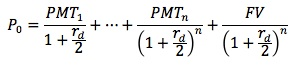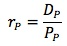### Why should I choose AnalystNotes?

AnalystNotes specializes in helping candidates pass. Period.

##### Subject 2. Cost of Debt and Preferred Stock

Capital components are the types of capital used by firms to raise fund. They include the items on the right side of a firm's balance sheet (debt, preferred stock, and common equity). Any increase in the firm's total assets must be financed by one or more of these capital components.

The cost of debt is defined as the cost to the firm in terms of the interest rate that it pays for ordinary debt (rd) less the tax savings that are achieved. Interest on debt is tax-deductible and therefore to calculate the cost of debt the tax benefit is deducted.

Two methods to estimate the before-tax cost of debt (rd) are discussed.

#### Yield-to-Maturity Approach

This approach uses the familiar bond valuation equation. Assuming semi-annual coupon payments, the equation is:The six-month yield (rd/2) is derived and then annualized to arrive at the before-tax cost of debt, rd.

#### Debt-Rating Approach

This approach can be used if there isn't a reliable market price for a firm's debt. Based on the company's debt rating, the before-tax cost of debt is estimated by using the yield on comparably rated bonds for maturities that are a close match to those of the firm's existing debt.

For example, assume that:

• A firm's debt has an average maturity of 5 years.
• Its credit rating is AAA.
• The yield on debt with the same debt rating and similar maturity is 6%.
• The marginal tax rate is 30%.

Then the company's after-tax cost of debt is 6% x (1 - 30%) = 4.2%.

Other factors, such as debt seniority and security, may complicate the calculation, so analysts must take care when determining the comparable debt rating and yield.

#### Issues in Estimating the Cost of Debt

• Fixed-rate debt versus floating-rate debt

Estimating the cost of floating-rate debt is difficult because the cost depends not only on the current yield but also on the future yields. The term structure of interest rates may be used to calculate an average rate.

• Debt with option-like features

Be aware that some debt can have call or put options. (Valuing such debts is a topic for Level II candidates.)

• Non-rated debt

The yields of a firm's debt may not be available, or a firm may not have rated bonds.

• Leases

If a company uses leasing as a source of capital, the cost of these leases should be included in the cost of capital (long-term debt).

#### Cost of Preferred Stock

The cost of preferred stock is calculated by dividing the dollar amount of the dividend (which is normally paid on an annual basis) by the preferred stock current price.It is important to note that tax does not affect the calculation of the cost of preferred stock, since preferred dividends are not tax deductible.

User Comment
DariSH Does anyone knows, shall we include operating lease into cost of debt, or is it just for capital leases?
czar it would be just capital leases.
operation leases go into the the Income stmt, not in the balance sheet.
hope this helps
gulfa99 Czar, wats an operational lease? Is it short term leasing.
jonan203 an operating lease would be an firm renting a building for a temporary period, in an operating lease you cannot realize any depreciation and it would be recognized as an expense

if the same firm leased the building with the intention of eventually owning it, it is classified as a capital lease and the firm can depreciate the asset and would be recognized on the balance sheet
Boydbri1 From the analyst perspective an operating lease should be included in the cost. This is a way for companies to hide debt and is therefore up to the analyst's discretion when comparing to other companies.
SWHY0104 generally should treat operating lease as debt. Moody's and S&P publish their methodologies for how to adjust debt for op leases. Look it up if interested
fobucina Yeah, you have to look to include operating leases as part of debt when valuing companies. Generally, companies provide in their statements a payment schedule for their operating leases and your task is to find the discounted value of those impending payments. If I'm not mistaken, the company's cost of debt is used to discount the pmts.
hirokeee Could someone explain why the price of preferred stock is the preferred dividends paid out divided by the current price (rather than price at issue? I.e., initial cash inflows to the issuer).